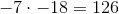## Example Questions

### Example Question #1 : How To Multiply Integers

The product of two consecutive positive integers is 272. What is the larger of the two integers?

16

19

18

17

15

17

Explanation:

In order to multiply to 272, the units digits of the two integers will have to multiply to a number with a units digit of 2. For 17, you can see that 6 x 7 (the units digits of 16 and 17) = 42. The best strategy here is to plug in the choices using the units digit strategy.

### Example Question #122 : Integers

What is the units digit of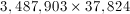?Explanation:

The units digit of any product depends on the units digits of the 2 numbers multiplied, which in this case is 3 and 4. Since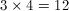, the units digit ofis 2.

### Example Question #123 : Integers

What is the units digit of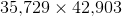Explanation:

The units digit of the product of any two numbers is the same as the units digit of the product of the two numbers' units digits. In this case, it would be the units digit of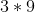, which is.

### Example Question #124 : Integers

Choose the answer below which best solves the following equation: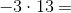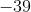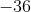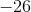Explanation:

When multiplying integers, if one of the integers is negative, your answer will be negative:

First multiply the ones digits together.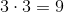Next multiply the ones digit of the smaller number with the tens digit of the larger number to get the tens digit of the product.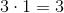Combining these two and remembering the negative sign we get our final answer: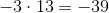### Example Question #191 : Arithmetic

Choose the answer which best solves the following equation: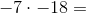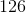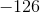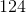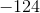Explanation:

When multiplying integers, if both of the signs of the integers are the same (positive, or negative) then your result will be positive:

First multiply the ones digit of both numbers together. If the product is greater than ten remember to carry the one to the tens place.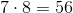Next multiply the ones digit of the smaller number with the tens digit of the larger number and add the number that was carried over.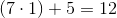Combine these two together to get: